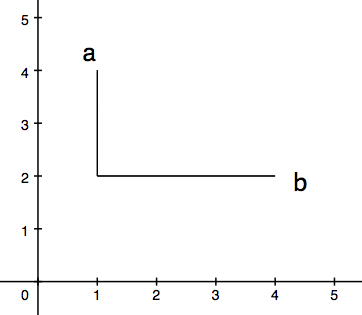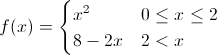Learning Calculus: Basics & Homework Help Final Exam

Free Practice Test Instructions:

Choose your answer to the question and click 'Continue' to see how you did. Then click 'Next Question' to answer the next question. When you have completed the free practice test, click 'View Results' to see your results. Good luck!

Answered 0 of 15

Question 1 1. Find the shortest distance between a and b by drawing a line connecting them and using the Pythagorean Theorem.Question 13 13. Consider the region bound by the x-axis, the y-axis, and f(x) between 0 and 4. What is an equation for the area of this region?Question 15 15. Consider the region bound by the x-axis, the y-axis, and f(x) between 0 and 4. What is the area of this region?Are you a student or a teacher?

Learning Calculus: Basics & Homework Help Final Free Practice Test Instructions

Choose your answer to the question and click 'Continue' to see how you did. Then click 'Next Question' to answer the next question. When you have completed the free practice test, click 'View Results' to see your results. Good luck!

Support